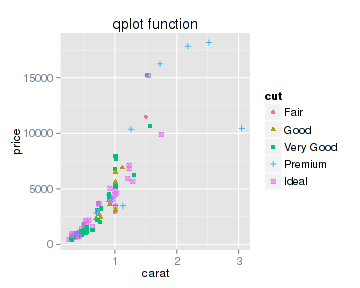# ggplot2作图详解1：入门函数qplot

`install.packages("ggplot2")`

### 1 qplot函数参数

```qplot(x, y = NULL, ..., data, facets = NULL,
margins = FALSE, geom = "auto", stat = list(NULL),
position = list(NULL), xlim = c(NA, NA),
ylim = c(NA, NA), log = "", main = NULL,
xlab = deparse(substitute(x)),
ylab = deparse(substitute(y)), asp = NA)```
• x, y： 意义明确，不用说了
• data： 这个可以有，为数据框（data.frame）类型；如果有这个参数，那么x，y的名称必需对应数据框中某列变量的名称
• facets： 图形/数据的分面。这是ggplot2作图比较特殊的一个概念，它把数据按某种规则进行分类，每一类数据做一个图形，所以最终效果就是一页多图
• margins： 是否显示边界
• geom： 图形的几何类型（geometry），这又是ggplot2的作图概念。ggplot2用几何类型表示图形类别，比如point表示散点图、line表示曲线图、bar表示柱形图等。
• stat： 统计类型（statistics），这个更加特殊。直接将数据统计和图形结合，这是ggplot2强大和受欢迎的原因之一。
• position： 图形或者数据的位置调整，这不算太特殊，但对于图形但外观很重要
• xlim, ylim, xlab, ylab, asp： 初步可以按照plot函数的相应参数来理解

### 2 qplot做散点图

#### 2.1 使用向量数据

```library(ggplot2)
x <- 1:1000
y <- rnorm(1000)
plot(x, y, main="Scatter plot by plot()")
qplot(x,y, main="Scatter plot by qplot()")```

#### 2.2 使用数据框数据

```str(diamonds)
## 'data.frame':	53940 obs. of  10 variables:
##  \$ carat  : num  0.23 0.21 0.23 0.29 0.31 0.24 0.24 0.26 0.22 0.23 ...
##  \$ cut    : Ord.factor w/ 5 levels "Fair"<"Good"<..: 5 4 2 4 2 3 3 3 1 3 ...
##  \$ color  : Ord.factor w/ 7 levels "D"<"E"<"F"<"G"<..: 2 2 2 6 7 7 6 5 2 5 ...
##  \$ clarity: Ord.factor w/ 8 levels "I1"<"SI2"<"SI1"<..: 2 3 5 4 2 6 7 3 4 5 ...
##  \$ depth  : num  61.5 59.8 56.9 62.4 63.3 62.8 62.3 61.9 65.1 59.4 ...
##  \$ table  : num  55 61 65 58 58 57 57 55 61 61 ...
##  \$ price  : int  326 326 327 334 335 336 336 337 337 338 ...
##  \$ x      : num  3.95 3.89 4.05 4.2 4.34 3.94 3.95 4.07 3.87 4 ...
##  \$ y      : num  3.98 3.84 4.07 4.23 4.35 3.96 3.98 4.11 3.78 4.05 ...
##  \$ z      : num  2.43 2.31 2.31 2.63 2.75 2.48 2.47 2.53 2.49 2.39 ...```

```set.seed(1000) # 设置随机种子，使随机取样具有可重复性
datax<- diamonds[sample(53940, 100), seq(1,7)]
##       carat   cut color clarity depth table price
## 17686  1.23 Ideal     H     VS2  62.2    55  7130
## 40932  0.30 Ideal     E     SI1  61.7    58   499
## 6146   0.90  Good     H     VS2  61.9    58  3989
## 37258  0.31 Ideal     G    VVS1  62.8    57   977```

```plot(x=datax\$carat, y=datax\$price, xlab="Carat", ylab="Price", main="plot function")
qplot(x=carat, y=price, data=datax, xlab="Carat", ylab="Price", main="qplot function")```

```plot(x=datax\$carat, y=datax\$price, xlab="Carat", ylab="Price", main="plot function", type='n')
cut.levels <- levels(datax\$cut)
cut.n <- length(cut.levels)
for(i in seq(1,cut.n)){
subdatax <- datax[datax\$cut==cut.levels[i], ]
points(x=subdatax\$carat, y=subdatax\$price, col=i, pch=i)
}
legend("topleft", legend=cut.levels, col=seq(1,cut.n), pch=seq(1,cut.n), box.col="transparent", cex=0.8)```

`qplot(x=carat, y=price, data=datax, color=cut, shape=cut, main="qplot function")````theme_set(theme_bw())
qplot(x=carat, y=price, data=datax, color=cut, shape=cut, main="qplot function")```

```qplot(x=carat, y=price, data=datax, shape=depth)
## Error: A continuous variable can not be mapped to shape```

### 3qplot做曲线图

```qplot(x=carat, y=price, data=datax, color=cut, geom="line", main="geom=\"line\"")
qplot(x=carat, y=price, data=datax, color=cut, geom=c("line", "point"), main="geom=c(\"line\", \"point\")")```

### 4 qplot做统计图

qplot是名副其实的qplot（quick plot）函数，通过改变几何类型geom参数的值你可以获得各种图形。geom参数可以设置的值和意义是：

• point：散点图
• line：曲线图
• smooth：平滑曲线
• jitter：另一种散点图
• boxplot：箱线图
• histogram：直方图
• density：密度分布图
• bar：柱状图

```qplot(carat, price, data = diamonds, color=cut, geom = "smooth", main = "smooth")
qplot(cut, price, data = diamonds, fill=cut, geom = "boxplot", main = "boxplot")
qplot(price, data = diamonds, fill=cut, geom = "histogram", main = "histogram")
qplot(price, data = diamonds, color=cut, geom = "density", main = "density")```

```qplot(price, data = diamonds, color=cut, geom = c("point", "density"))
## Error: geom_point requires the following missing aesthetics: y```

### 5 qplot做柱形图

```qplot(x=cut, y=price, data = diamonds, fill=cut, geom = "histogram",
stat="summary", fun.y="mean")```

stat参数表示统计的类型，而fun.y则表示应用于统计的函数。把geom参数值换成bar得到相同的图形：

```qplot(x=cut, y=price, data = diamonds, fill=cut, geom = "bar",
stat="summary", fun.y="mean")```

```(mean.price <- with(diamonds, aggregate(price~cut, FUN=mean)))
##         cut price
## 1      Fair  4359
## 2      Good  3929
## 3 Very Good  3982
## 5     Ideal  3458
qplot(x=cut, y=price, data=mean.price, fill=cut, geom="bar", stat="identity")```

### 6 SessionInfo

```sessionInfo()
## R version 3.1.0 (2014-04-10)
## Platform: x86_64-pc-linux-gnu (64-bit)
##
## locale:
##   LC_CTYPE=zh_CN.UTF-8       LC_NUMERIC=C
##   LC_TIME=zh_CN.UTF-8        LC_COLLATE=zh_CN.UTF-8
##   LC_MONETARY=zh_CN.UTF-8    LC_MESSAGES=zh_CN.UTF-8
##   LC_PAPER=zh_CN.UTF-8       LC_NAME=C
##  LC_MEASUREMENT=zh_CN.UTF-8 LC_IDENTIFICATION=C
##
## attached base packages:
##  tcltk     stats     graphics  grDevices utils     datasets  methods
##  base
##
## other attached packages:
##  mgcv_1.7-29     nlme_3.1-117    ggplot2_0.9.3.1 zblog_0.1.0
##  knitr_1.5
##
## loaded via a namespace (and not attached):
##   colorspace_1.2-4 digest_0.6.4     evaluate_0.5.3   formatR_0.10
##   grid_3.1.0       gtable_0.1.2     highr_0.3        labeling_0.2
##   lattice_0.20-29  MASS_7.3-31      Matrix_1.1-3     munsell_0.4.2
##  plyr_1.8.1       proto_0.3-10     Rcpp_0.11.1      reshape2_1.2.2
##  scales_0.2.4     stringr_0.6.2    tools_3.1.0```

• 本文由 发表
• 转载请务必保留本文链接：https://www.plob.org/article/7452.html